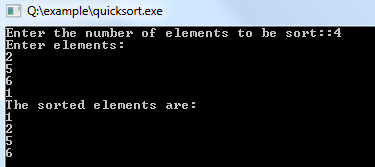# Quick Sort Algorithm

Quick sort is one of the most famous sorting algorithms based on divide and conquers strategy which results in an O(n log n) complexity. So, the algorithm starts by picking a single item which is called pivot and moving all smaller items before it, while all greater elements in the later portion of the list. This is the main quick sort operation named as a partition, recursively repeated on lesser and greater sublists until their size is one or zero - in which case the list is wholly sorted. Choosing an appropriate pivot, as an example, the central element is essential for avoiding the severely reduced performance of O(n2).

### Algorithm for Quick Sort

1. algorithm Quick_Sort(list)
2. Pre: list 6= fi
3. Post: the list has been sorted in ascending order
4. if list.Count = 1 // list already sorted
5. return list
6. end if
7. pivot <- Median_Value(list)
8. for i <- 0 to list.Count - 1
9. if list[i] = pivot
10. equal.Insert(list[i])
11. end if
12. if list[i] < pivot
13. less.Insert(list[i])
14. end if
15. if list[i] > pivot
16. greater.Insert(list[i])
17. end if
18. end for
19. return Concatenate(Quick_Sort(less), equal, Quick_Sort(greater))
20. end Quick_sort

Example:

``````#include <iostream>
#include <stdio.h>
template <class t>
using namespace std;

void quick_sort(t a[], int low, int high)
{
t k;
int i, j, flag = 1;
if (low < high) {
k = a[low];
i = low + 1;
j = high;
while (flag) {
while ((a[i] <= k) && (i < j)) i++; while (a[j] > k)
j--;
if (i < j)
swap(a, i, j);
else
flag = 0;
}
swap(a, low, j);
quick_sort(a, low, j - 1);
quick_sort(a, j + 1, high);
}
}

template
void swap(t1 a[], int x, int y)
{
t1 temp;
temp = a[x];
a[x] = a[y];
a[y] = temp;
}
int main()
{
int i, n, a;
cout << "Enter the number of elements to be sort::"; cin >> n;
cout << "Enter elements:\n";
for (i = 0; i < n; i++) cin >> a[i];
quick_sort(a, 0, n - 1);
cout << "The sorted elements are:\n";
for (i = 0; i < n; i++)
cout << a[i] << endl;
;
}
``````

Output: